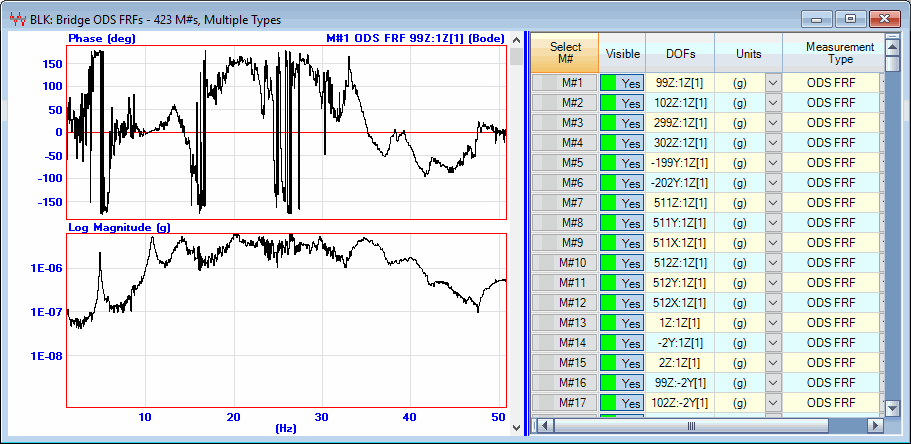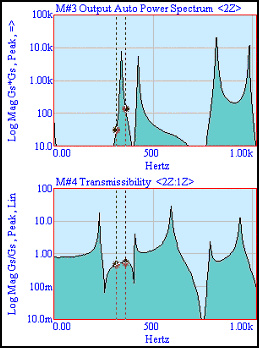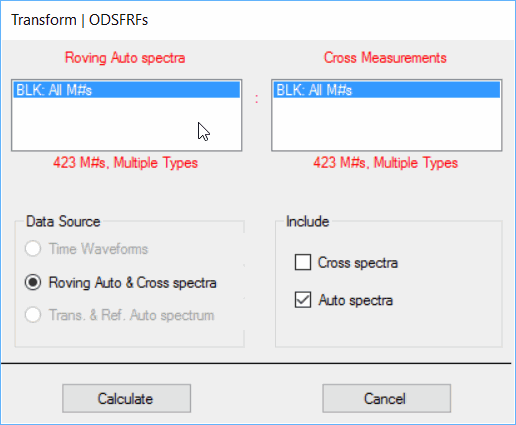# Transform | ODSFRFs

Calculates a set of ODSFRFs from operational (or output-only) data.

## What is an ODSFRF?

• An ODSFRF is formed by combining the Auto spectrum of each Roving response with the phase of the Cross spectrum between the Roving response and a (fixed) Reference response.

• An ODSFRF is complex valued frequency domain function that is like an FRF.A Typical ODSFRF.

1. The ODSFRF provides the true response (in displacement, velocity or acceleration units) at each DOF of a machine or structure, together with its phase relative to a Reference response.

2. An ODSFRF contains peaks at resonant frequencies.

3. ODS's cab be displayed  in animation from set of ODSFRFs

4. Operating mode shapes. can be extracted by curve fitting a set of ODSFRFs

## ODS's from a Set of ODSFRFs

If shape data from the cursor position in two or more ODSFRFs is displayed in animation on a model of a test structure, the resulting ODS is the true overall response of the structure at each DOF, with the correct phase relative to all other DOF.

## What is Transmissibility?

Transmissibility is defined as the spectrum of a Roving response divided by the spectrum of a Reference response.  It is the traditional measurement that is calculated when no excitation forces are measured.  Transmissibility is calculated in the same way as an FRF, but a (fixed) Reference response is used instead of the unmeasured force.

An advantage of the Transmissibility is that if the excitation force level varies from one Measurement Set to the next during data acquisition, its effect on both the Roving and Reference responses is assumed to be the same, so its effect will be "canceled out" in the Transmissibility calculation.

## Difficulty with Transmissibility's

A Transmissibility has a ”flat spot” instead of a peak in the vicinity of each resonant frequency, as shown below.Roving Auto Spectrum & Transmissibility.

## Scaled Cross Spectra

• If a set of Transmissibility's is multiplied by a single Reference response Auto spectrum, the resulting set of Scaled Cross Spectra can be used like a set of ODSFRFs.

• A set Cross Spectra does not have to be re-scaled, even if the excitation force level changes between Measurement Sets.

Scaled Cross Spectrum = Transmissibility x Reference Auto Spectrum

## Advantages of Scaled Cross Spectra

• A scaled Cross Spectrum contains peaks at resonant frequencies.

• No re-scaling is required if multiple Measurement Sets of data are used.

• A set of Scaled Cross Spectra can be curve fit to obtain operating mode shapes.

## Operating Mode Shapes

• At or near a resonant frequency, the ODS obtained either from a set of ODSFRFs or Scaled Cross Spectra is often dominated by the mode shape of that resonance.

• A set of ODSFRFs or Scaled Cross Spectra can be curve fit to obtain operating modal parameters (frequency, damping, and mode shape) for each resonance.

The Transform | ODSFRFs command can process multiple Measurement Sets of three different types of Source data;

• Multiple time domain Roving responses, plus a Reference time domain response.

• Roving response Auto spectra, plus Cross spectra between each Roving response and a Reference response.

• Transmissibility's between each Roving response and a Reference response, plus the Reference response Auto spectrum.

To calculate ODSFRFs from time waveforms,

• All Roving response M# must be defined as Outputs in the Input Output column of the M#s spreadsheet.

• All Reference response M# must be defined as Inputs in the Input Output column of the M#s spreadsheet.

• Each M# should contain the DOF (point & direction) at which it was acquired.

To calculate ODSFRFs from Auto & Cross spectra,

• All Auto spectrum M#s must be defined as Outputs in the Input Output column of the M#s spreadsheet.

• All Cross spectrum M#s must be defined as Cross M#s in the Input Output column of the M#s spreadsheet.

• Each Auto spectrum DOF must match the Roving DOF of a Cross spectrum.

To calculate Scaled Cross Spectra from multiple Measurement Sets of Transmissibility data,

• Each Measurement Set must include the same an Auto spectrum for the same Reference response, defined as an Input in the Input Output column of the M#s spreadsheet.

• All Transmissibility M#s must be defined as Cross M#s in the Input Output column of the M#s spreadsheet.

• Each Transmissibility Reference DOF must match the Roving DOF of the Auto spectrum of the Reference response for that Measurement Set.

When this command is executed, the following dialog box is opened.NOTE:  The Source measurements can be stored either in the same Data Block, or in two different Data Blocks.

## Measurement Sets

• If the M# DOFs also contain [Measurement Set] numbers, then all data in each Measurement Set is processed independently of the other Measurement Sets.

• If the Reference Auto spectra are different between Measurement Sets (indicating the force levels have changed), the ODSFRFs must be re-scaled using the Transform | Scale ODSFRFs command.